# MP4: IIR Notch Filtering¶

Audio recordings sometimes have 60Hz hum noise, caused by leaving the microphone cable to close to a power line. This MP will explore methods for getting rid of the hum noise, especially the low-frequency harmonics. This MP was originally designed by Prof. Doug Jones at the University of Illinois. The code package can be downloaded from http://courses.engr.illinois.edu/ece401/fa2020/ece401_20fall_mp4.zip.

In :
import numpy as np
import matplotlib.pyplot as plt
import matplotlib.figure
import wave
%matplotlib inline

In :
import importlib
import mp4

Out:
<module 'mp4' from '/Users/jhasegaw/Dropbox/mark/teaching/ece401/ece401labs/20fall/mp4/src/mp4.py'>

## Exploring the Data¶

This MP will explore one audio recording in detail, so that you can see where the low-frequency peaks are. The main peak, at 120Hz, could easily be filtered out from a large number of audio files, all at once.

In :
with wave.open('input.wav','rb') as w:
fs = w.getframerate()
nsamps = w.getnframes()

In :
t = np.linspace(0,nsamps/fs,nsamps)
fig=plt.figure(figsize=(14,4))
ax=fig.subplots()
ax.plot(t[(0.5<t)&(t<1.1)],x[(0.5<t)&(t<1.1)])

Out:
[<matplotlib.lines.Line2D at 0x7fb3db30da00>]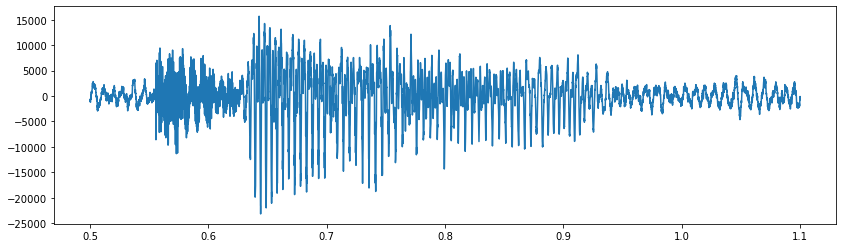Notice, in the waveform above, there is a periodic component in the "silence" before and after the speech begins. There seem to be five or six periods in 0.05sec, so maybe the period is around 0.01sec, or around 100Hz.

## How to debug¶

Rather than printing every plot using separate blocks for both the distributed solution and your solution, instead, each of the following blocks will include lines to plot either one or the other. In order to switch between your solution and the distributed solution, you'll need to comment out the other one.

In :
import h5py
solutions = h5py.File('solutions.hdf5','r')


## Searching for the noise peaks¶

First, let's plot a spectrum, and try to find the frequencies of the noise components.

In :
importlib.reload(mp4)
#spec=mp4.todo_spectrum(x)
spec = solutions['spec'][:]
f = np.linspace(0,fs,nsamps,endpoint=False)
fig=plt.figure(figsize=(14,4))
ax=fig.subplots()
ax.plot(f[f<500],spec[f<500])

Out:
[<matplotlib.lines.Line2D at 0x7fb3db829730>]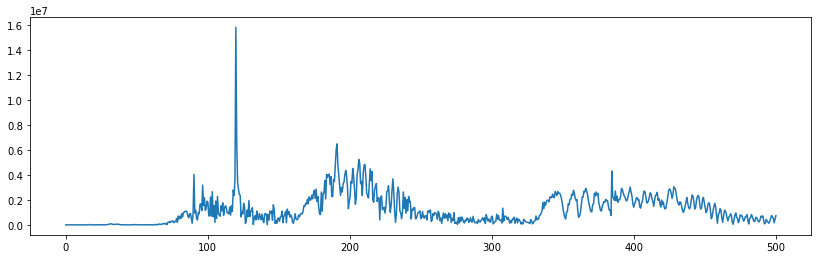• It looks like there's a huge power-line noise component at about 120Hz.
• There doesn't seem to be any 60Hz component; the recording device seems to have filtered out all energy below about 80Hz.
• There is also energy at about 180Hz, which might be the third harmonic of the power line noise, but it might also be the pitch of a female talker. Let's leave that energy in the signal.
• Instead, let's eliminate three of the other small peaks that occur between 90Hz and 120Hz.
• We'll use the "todo_findpeak" function to find the frequencies of those peaks (if you've written it).
In :
importlib.reload(mp4)
#noise1 = mp4.todo_findpeak(spec, fs, 0, 100)
#noise2 = mp4.todo_findpeak(spec, fs, noise1+1, 100)
#noise3 = mp4.todo_findpeak(spec, fs, 100, 110)
#noise4 = mp4.todo_findpeak(spec, fs, 100, 150)
#freqs = np.array([noise1,noise2,noise3,noise4])
freqs = solutions['freqs'][:]
print(freqs)

[ 90.28444206  96.42624084 103.18221949 119.7650762 ]


## Creating the notch filters¶

We'll create four notch filters: one for each of the frequencies we want to eliminate. For each notch filter, we need to create zeros and poles for the Z transform, then convert them to filter coefficients.

In :
importlib.reload(mp4)
#z = mp4.todo_zeros(freqs,fs)
z = solutions['z'][:]
z

Out:
array([[0.99937155+0.03544719j, 0.99928315+0.03785745j,
0.99917919+0.04050848j, 0.99889422+0.0470143j ],
[0.99937155-0.03544719j, 0.99928315-0.03785745j,
0.99917919-0.04050848j, 0.99889422-0.0470143j ]])
In :
importlib.reload(mp4)
#p = mp4.todo_poles(z,20,fs)
p = solutions['p'][:]
np.log(np.abs(p))

Out:
array([[-0.000625, -0.000625, -0.000625, -0.000625],
[-0.000625, -0.000625, -0.000625, -0.000625]])
In :
def pole_zero_plot(z,p):
fig=plt.figure(figsize=(10,8))
ax=fig.subplots()
ax.plot([0,1e-6],[-2,2],'k-',[-2,2],[0,0],'k-')  # plot the axes
omega = np.linspace(0,2*np.pi,200)
ax.plot(np.cos(omega),np.sin(omega),'k-') # plot the unit circle
ax.set_aspect('equal') # make it look like a circle
zeromarker = matplotlib.markers.MarkerStyle(marker='o',fillstyle='none')
polemarker = matplotlib.markers.MarkerStyle(marker='x',fillstyle='none')
ax.scatter(x=np.real(z),y=np.imag(z),s=40,marker=zeromarker)
ax.scatter(x=np.real(p),y=np.imag(p),s=40,marker=polemarker)
return(ax)
ax=pole_zero_plot(z,p)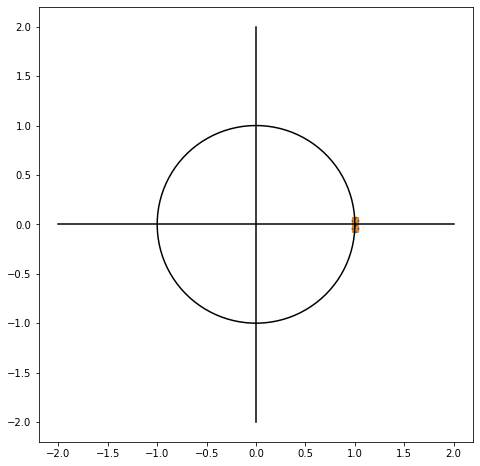At that scale, the poles and zeros are all right on top of each other! Let's try to zoom in.

In :
ax=pole_zero_plot(z,p)
ax.set_xlim([0.95,1.05])
ax.set_ylim([-0.05,0.05])

Out:
(-0.05, 0.05)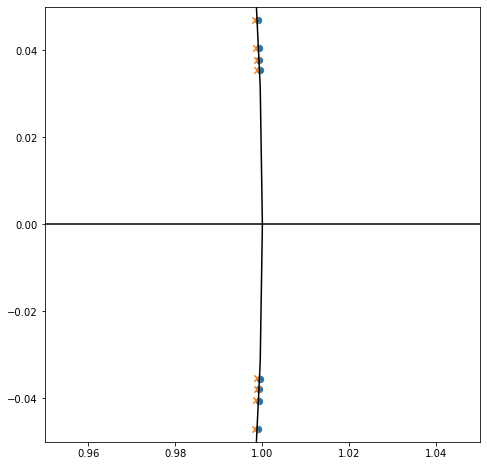Finally, let's create the filter coefficients. There are four filters, each of which has feedforward coefficients (b[:,k]) and feedback coefficients (a[:,k]).

In :
importlib.reload(mp4)
#a = mp4.todo_coefficients(p)
a = solutions['a'][:]
#b = mp4.todo_coefficients(z)
b = solutions['b'][:]
fig = plt.figure(figsize=(10,8))
axs=fig.subplots(len(freqs),2)
for row in range(len(freqs)):
axs[row,0].stem(b[:,row],use_line_collection=True)
axs[row,0].set_title('Feedforward coefficients, filter %d'%(row))
axs[row,1].stem(a[:,row],use_line_collection=True)
axs[row,1].set_title('Feedback coefficients, filter %d'%(row))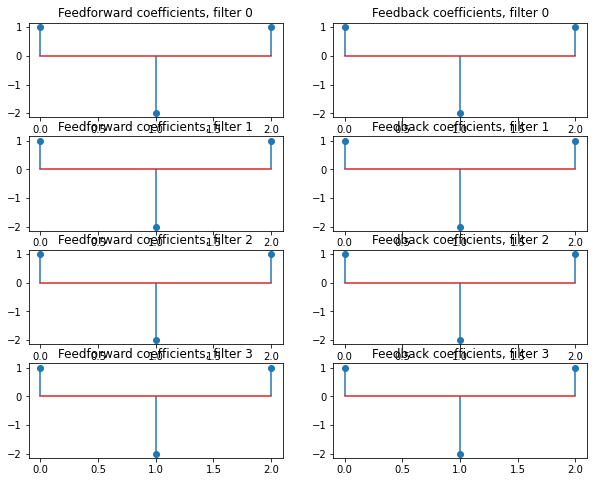Wow. All eight sets of coefficients are almost identical. Just to make sure they're not exactly identical, let's print them out.

In :
print(b,a)

[[ 1.          1.          1.          1.        ]
[-1.9987431  -1.9985663  -1.99835839 -1.99778843]
[ 1.          1.          1.          1.        ]] [[ 1.          1.          1.          1.        ]
[-1.99749428 -1.99731759 -1.99710981 -1.99654021]
[ 0.99875078  0.99875078  0.99875078  0.99875078]]


## Filtering out the noise¶

Let's plot the frequency response that we would get by running all four filters, one after the other.

In :
importlib.reload(mp4)
#H = np.ones(nsamps,dtype='complex')
#for k in range(len(freqs)):
#omega, Hnew = mp4.todo_freqresponse(a[:,k],b[:,k],nsamps)
#H *= Hnew
H = solutions['H'][:]
fig=plt.figure(figsize=(14,4))
ax=fig.subplots()
plt.plot(f[f<400],np.abs(H[f<400]))

Out:
[<matplotlib.lines.Line2D at 0x7fb3dc0d50d0>]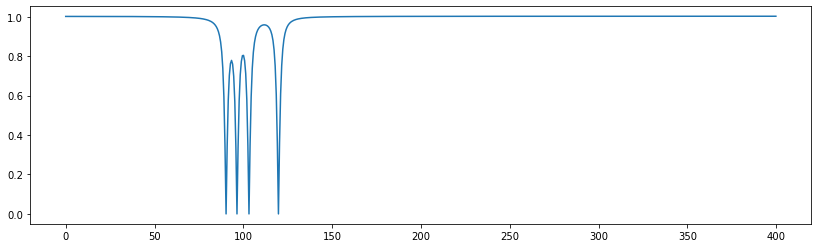OK, now let's actually run all four filters, one after the other, and take a look at the resulting spectrum and signal, to see if the noise has been removed.

In :
importlib.reload(mp4)
#y = x
#for k in range(len(freqs)):
#    y = mp4.todo_filter(y,a[:,k],b[:,k])
y = solutions['y']
yspec=mp4.todo_spectrum(y)
fig=plt.figure(figsize=(14,4))
ax=fig.subplots()
ax.plot(f[f<500],yspec[f<500])

---------------------------------------------------------------------------
NotImplementedError                       Traceback (most recent call last)
<ipython-input-17-b1f53d57a430> in <module>
4 #    y = mp4.todo_filter(y,a[:,k],b[:,k])
5 y = solutions['y']
----> 6 yspec=mp4.todo_spectrum(y)
7 fig=plt.figure(figsize=(14,4))
8 ax=fig.subplots()

~/Dropbox/mark/teaching/ece401/ece401labs/20fall/mp4/src/mp4.py in todo_spectrum(x)
9     '''
10     spec = np.zeros(len(x),dtype='complex')
---> 11     raise NotImplementedError('You need to write this part!')
12     return(np.abs(spec))
13

NotImplementedError: You need to write this part!
In :
t = np.linspace(0,nsamps/fs,nsamps)
fig=plt.figure(figsize=(14,4))
ax=fig.subplots()
ax.plot(t[(0.5<t)&(t<1.1)],y[(0.5<t)&(t<1.1)])

Out:
[<matplotlib.lines.Line2D at 0x7fb3dc5f17f0>]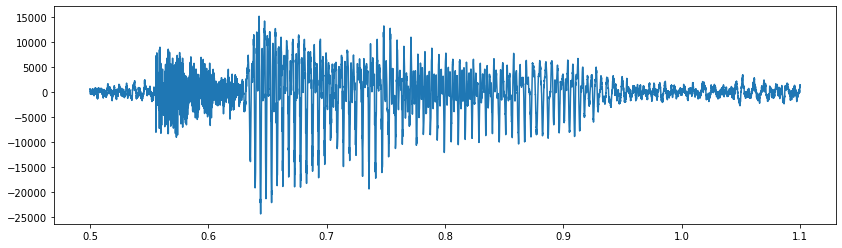## Conclusion¶

That's all! This is the basic technology behind utilities like noiseremove, in case you don't feel like doing it all by hand next time.

In [ ]: